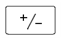## Signed Numbers Assignment Help

Assignment Help: >> Introductory Mathematics - Signed Numbers

Signed Numbers:

Chapter signed number covers the processes of addition, subtraction, division & multiplication of signed numbers.

APPLIES one of the arithmetic operations of addition, subtraction, multiplication & division using signed numbers.

Calculator Usage, Special Keys

Change Sign keyPressing this key changes the sign of the number in the above display. For enter a negative number, the number is entered as a positive number and then the change sign key is pressed to convert it to a negative. The display will show a "-" in front of the number.

 Addition Division Multiplication Subtraction#### Assured A++ Grade

Get guaranteed satisfaction & time on delivery in every assignment order you paid with us! We ensure premium quality solution document along with free turntin report!